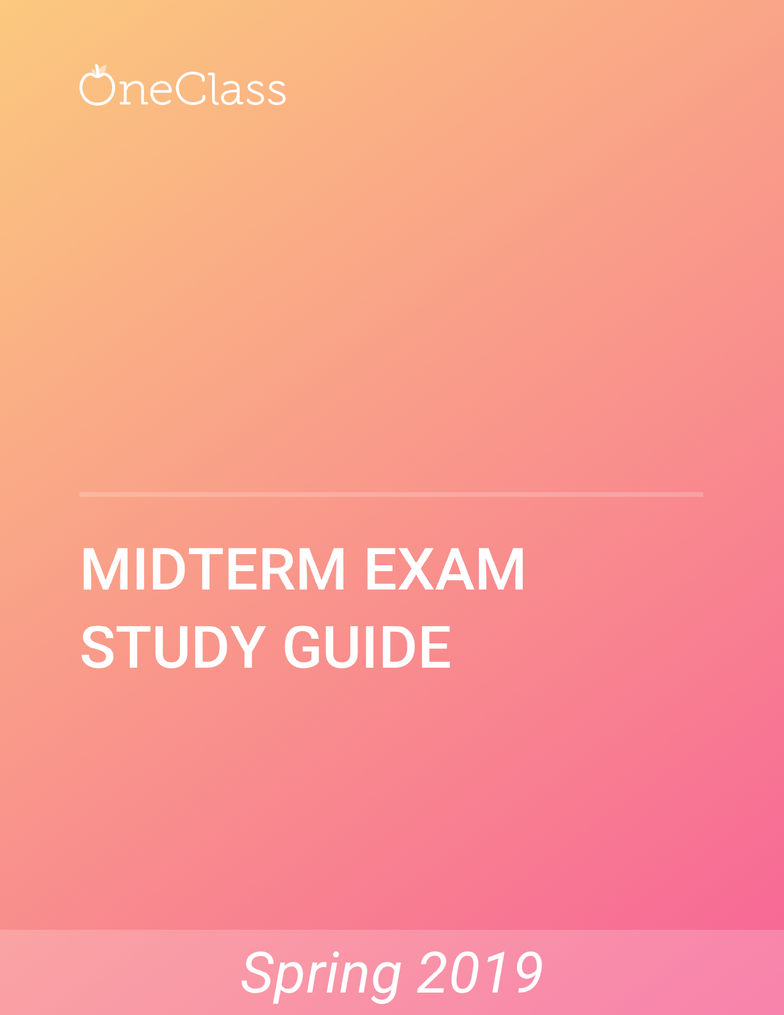Study Guides (400,000)
US (230,000)
NCSU (600)
MA (20)
MA 108 (2)
Study Guide

# MA 108 Study Guide - Spring 2019, Comprehensive Final Exam Notes - Triangle, The Matrix, Positive-Definite Matrix

Department
Mathematics
Course Code
MA 108
Professor
Elyse Suzanne Rogers
Study Guide
Final

This preview shows pages 1-3. to view the full 23 pages of the document.MA 108

Only pages 1-3 are available for preview. Some parts have been intentionally blurred.Only pages 1-3 are available for preview. Some parts have been intentionally blurred.5) Compositions: When two or more transformations are combined to produce a single
transformation, the result is called a composition of the transformations. The composition of
two or more isometries is also an isometry. Compositions may preserve or reverse orientation
depending on how many reflections they contain.
An important type of composition is a glide reflection, which is a translation followed by
a reflection in a line parallel to the direction of the translation. A glide reflection is an isometry
that reverses the orientation of figures. For example, suppose a translation 6 units to the right is
followed by a reflection in the x-axis. This glide reflection can be written in coordinate form as
(x’,y’) = ( x + 6, -y ). The glide reflection ( x’, y’ ) = ( -x, y +4 ) is a translation 4 units up followed
by a reflection in the y-axis. The order in which two transformations are performed sometimes
affects the resulting image, but with a glide reflection the image would be the same regardless
of the order of the transformations (translation first or reflection first).
Try these: Write a brief description of each transformation:
1) ( x’, y’ ) = ( x - 3, -y ) a translation 3 units to the left, followed by a reflection in the x-axis.
2) ( x’, y’ ) = ( -x, y - 8 ) a translation 3 units down, followed by a reflection in the y-axis.
3) ( x’, y’ ) = ( y + 2, x + 2 ) a translation 2 units up, and 2 units to the right.
4) ( x’, y’ ) = ( x + 10, -y ) a translation 10 units to the right, followed by a reflection in the x-axis.
5) ( x’, y’ ) = ( -x, y + 6 )a translation 6 units up, followed by a reflection in the y-axis.
Write each transformation in coordinate form:
6) Translation 5 units right and 5 units up, reflection in the line y = x. ( x’, y’ ) = ( x+5, y+5) y,x
7) Translation 8 units left, reflection in the x-axis. ( x’, y’ ) = ( x-8, -y )
8) Translation 5 units down, reflection in the y-axis. ( x’, y’ ) = (- x, y-5 )
9) Translation 4 units right, reflection in the x-axis.( x’, y’ ) = (x+4, -y)
Some transformations can be thought of as a composition of two reflections. A
translation is the composition of two reflections in parallel lines. The direction of the translation
is perpendicular to the lines, and the distance translated is twice the distance between the lines.
A rotation can be expressed as the composition of two reflections in intersecting lines. The
center of the rotation is the point where the lines intersect, and the angle of the rotation is twice
the angle between the lines.
find more resources at oneclass.com
find more resources at oneclass.com

Unlock to view full version

Only pages 1-3 are available for preview. Some parts have been intentionally blurred.Compositions of reflections and rotations can be expressed as products of the 2x2
matrices used for the component transformations. For example, if we perform a 90-degree
rotation about the origin, followed by a reflection in the x-axis, then we can determine the overall
effect of this composition by multiplying the matrix that reflects in the x-axis by the matrix that
rotates a figure 90 degrees:
[ 1 0 [ 0 -1 = [ 0 -1
0 -1 ] 1 0 ] -1 0 ]
The product is a reflection in the line y = -x. If we reverse the order of the composition and do
the reflection first, we get:
[ 0 -1 [ 1 0 = [ 0 1
1 0 ] 0 -1 ] 1 0 ]
The product is a reflection in the line y = x. These examples show that the order in which the
transformations are performed can affect the result of the composition. When composing
rotations or reflections, make sure that the matrix for the first transformation is on the right, and
the matrix for the second transformation is on the left.
Try these: Use matrix multiplication to determine the net result of each composition:
1) Reflection in the line y = x, followed by a 270-degree rotation about the origin:
[ 0 1 [ 0 1 = [ 1 0
-1 0 ] 1 0 ] 0 -1 ] , which is the matrix for a reflection in the x-axis.
2) Rotation 270 degrees about the origin, followed by a reflection in the line y = x.
[ 0 1 [ 0 1 = [ -1 0
1 0 ] -1 0 ] 0 1 ]
3) Reflection in the y-axis, followed by a reflection in the line y = -x.
[ -1 0 [ 0 -1 = [ 0 1
0 1 ] -1 0 ] -1 0 ]
4) Rotation 180 degrees about the origin, followed by a reflection in the y-axis.
[ -1 0 [ -1 0 = [ 1 0
0 -1 ] 0 1 ] 0 -1 ]
5) Reflection in the y-axis, followed by a rotation of 180 degrees about the origin.
[ -1 0 [ -1 0 = [ 1 0
0 1 ] 0 -1 ] 0 1 ]
find more resources at oneclass.com
find more resources at oneclass.com